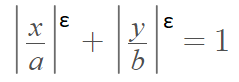# Supercircle and Subcircle

## What is a Supercircle?

A supercircle gets its definition directly from a superellipse. Superellipses were introduced by Gabriel Lamé in 1818. They can be defined by the implicit equationWhere a, b, and ε are positive constants. You might also see the exponent as r or n instead of ε.
Note: if x or y is negative, avoid imaginaries because the modulus signs can be fractions.

The formula can be rewritten as a function of x2, y2, and an exponent ε. When a = b = 1 1 (i.e., the semi-major and semi-minor axes are equal), the superellipse equation describes a unit radius supercircle:
(x2)ε + (y2)ε = 1.

The value for ε controls the shape .

• When ε = 1, the supercircle is a square,
• When ε > 1, the shape is convex outwards,
• When ε < 1, the shape is convex inwards.
• When ε = 2/3, it is an astroid.
• When ε = 2, it is a traditional circle.
• When ε = 4, it’s a squircle.

## Area of a Supercircle

The area of a supercircle can be found with the integralWe can show this works by using ε = 1, the unit circle. We know that the area of a unit circle = πr2 = π12 = π. Our integral for the unit circle becomes:which equals π.

## Subcircle

Generally speaking, a subcircle is defined as “not quite circular” . Strangely, the term could describe any of the “supercircles” defined above, as they aren’t quite circular. The term isn’t defined formally in mathematics, but you will find it as an option in many routines in machine learning and computer science. For example, you’ll find subcircle in the ‘soilprofile’ R package, where it describes a “Dataset of coordinates for a subcircle-shaped skeleton unit” .

You might also come across the option when processing LiDar data files. You can “thicken” your points as you thin them to simulate a laser beam’s diameter for the laser beam, creating a “nice set of points for subsequent CHM or DSM construction” .

## References

Graph: Created with Desmos.
 Machine Learning for Multimodal Interaction. First International Workshop, MLMI 2004, Martigny, Switzerland, June 21-23, 2004, Revised Selected Papers.
 Guha, S. (2014). Computer Graphics Through OpenGL: From Theory to Experiments. CRC Press.
 Mirriam Webster. Subcircular. Retrieved March 2, 2022 from: https://www.merriam-webster.com/dictionary/subcircular#:~:text=Definition%20of%20subcircular,nearly%20circular%20%3A%20not%20quite%20circular